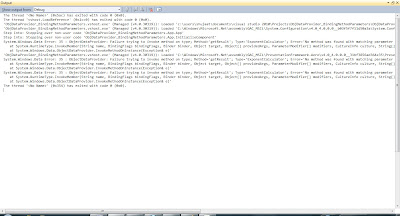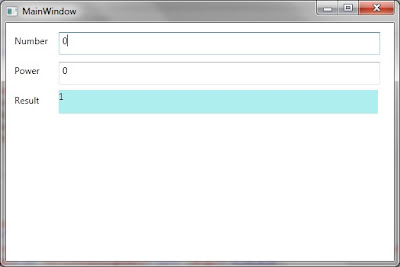## Tuesday, February 1, 2011

### WPF - Binding Method Parameters of ObjectDataProvider

In this post we will be discussing how we can use data returned by a method for WPF Binding in XAML using ObjectDataProvider. We will also be discussing how we can work around the requirement of WPF Binding to have a non-DependencyProperty based Binding target. Using non-Dependency properties as a Binding target. We will also shed some light on Binding to ObjectDataProvider instance instead of ObjectDataProvider.Data.

Let's create a sample WPF application ObjDataProvider_BindingMethodParameters. Add a class named ExponentCalculator. This class just has a public method getResult. This method receives two arguments. It just evaluates the second argument as exponent to the first argument and returns the result. It uses Pow static method of System.Math class.
`namespace ObjDataProvider_BindingMethodParameters{    using System;    class ExponentCalculator    {        public double getResult(double number, double exponent)        {            double result = Math.Pow(number, exponent);                                    return result;        }    }}`

Now we use the above class into action. We update the definition of MainWindow.xaml . We are using the same ObjectDataProvider as we have used in previous post. We are just using another feature of ObjectDataProvider. It can grab data from a method of a type. It also introduces a property Data which is used for Binding the data returned by the method.

It even allows to specify parameters to the method as you can see in the following code that we have specified two parameters. They are both of type double. We needed to add System namespace to use Double. We have specified it to use the alias sys which we are using to define types of method parameters.
`<Window x:Class="ObjDataProvider_BindingMethodParameters.MainWindow"        xmlns="http://schemas.microsoft.com/winfx/2006/xaml/presentation"        xmlns:x="http://schemas.microsoft.com/winfx/2006/xaml"        xmlns:sys="clr-namespace:System;assembly=mscorlib"        xmlns:local="clr-namespace:ObjDataProvider_BindingMethodParameters"        Title="MainWindow" Height="350" Width="525">    <Window.Resources>        <ObjectDataProvider ObjectType="{x:Type local:ExponentCalculator}"                             MethodName="getResult" x:Key="expCalculator">            <ObjectDataProvider.MethodParameters>                <sys:Double>4</sys:Double>                <sys:Double>2</sys:Double>            </ObjectDataProvider.MethodParameters>        </ObjectDataProvider>                    </Window.Resources>    <Grid>        <TextBox Height="30" HorizontalAlignment="Left" Margin="69,12,0,0"                  Name="textBoxNumber" VerticalAlignment="Top" Width="422"                  Text="{Binding Source={StaticResource expCalculator}, Path=MethodParameters, BindsDirectlyToSource=True, Mode=OneWayToSource}"/>        <Label Content="Number" Height="33" HorizontalAlignment="Left" Margin="6,10,0,0"               Name="label1" VerticalAlignment="Top" Width="57" />        <TextBox Height="30" HorizontalAlignment="Left" Margin="69,51,0,0" Name="textBoxPower"                  VerticalAlignment="Top" Width="422"                   Text="{Binding Source={StaticResource expCalculator}, Path=MethodParameters, BindsDirectlyToSource=True, Mode=OneWayToSource}"/>        <Label Content="Power" Height="33" HorizontalAlignment="Left" Margin="6,49,0,0"                Name="label2" VerticalAlignment="Top" Width="57" />        <Label Content="Result" Height="33" HorizontalAlignment="Left" Margin="6,88,0,0"               Name="label3" VerticalAlignment="Top" Width="57" />        <TextBlock Height="31" HorizontalAlignment="Left" Margin="69,88,0,0" Name="textBlockResult"                Background="PaleTurquoise"    VerticalAlignment="Top" Width="419" >            <TextBlock.Text>                <Binding Source="{StaticResource expCalculator}"  />            </TextBlock.Text>        </TextBlock>    </Grid></Window>`

Generally we have seen that Binding is defined on the Target element. But WPF has this requirement that the target properties must be dependency properties. So we can not define binding there. But we still want user to type some number in the two text boxes and get the result. In order to still fulfill the requirement, we are exploiting one of the WPF Binding mode, it is OneWayToSource. This is used for the Text properties of the two text boxes. Here Method parameter is considered as source and whatever data entered in the text boxes, would be available in MethodParameters collection.

Now when we Bind with an ObjectDataProvider, the binding takes place with Data property of ObjectDataProvider. WPF makes the data available in this property. Since Method Parameters are not from the Data itself how should we do that? Basically Binding allows this too. If we set BindsDirectlyToSource, it is bound to the ObjectDataProvider instance itself instead of the Data property of ObjectDataProvider. Since we need to show the data returned from the method, we are binding the ObjectDataProvider instance to the Text property of TextBlock using StaticResource markup extension. As we have discussed, this would bind to Data property of ObjectDataProvider instance.

Now lets run the application. It shows the MainWindow as follows:We enter some data in the text boxes but it seems no evaluation is being done as the TextBlock showing the result is empty. In order to determine what is actually going on lets update the Visual Studio settings to be more watchful of the binding by enabling more restrictive Trace Settings for WPF Binding. Go to Tool -> Options in Visual Studio and set Tracing for DataBinding to All.Now run the application and see the output window:Now we see what is going on. Basically the Text property of TextBox is of type string. We need to convert the text entered to double so that correct method can be called. Let us define an IValueConverter for this purpose.
`namespace ObjDataProvider_BindingMethodParameters{    #region namespaces    using System;    using System.Windows.Data;    #endregion namespaces    [ValueConversion(typeof(string), typeof(double))]    public class doubleStringConverter : IValueConverter    {        public object Convert(object value, Type targetType, object parameter, System.Globalization.CultureInfo culture)        {            //throw new NotImplementedException();            return value.ToString();        }        public object ConvertBack(object value, Type targetType, object parameter, System.Globalization.CultureInfo culture)        {            //throw new NotImplementedException();            double doubleValue = 0.0;            string strText = (value == null) ? string.Empty : value.ToString();            if (strText != string.Empty)            {                doubleValue = double.Parse(strText);            }            return doubleValue;        }     }}`

To use this converter, we update the view as follows:
`<Window x:Class="ObjDataProvider_BindingMethodParameters.MainWindow"        xmlns="http://schemas.microsoft.com/winfx/2006/xaml/presentation"        xmlns:x="http://schemas.microsoft.com/winfx/2006/xaml"        xmlns:sys="clr-namespace:System;assembly=mscorlib"        xmlns:local="clr-namespace:ObjDataProvider_BindingMethodParameters"        Title="MainWindow" Height="350" Width="525">    <Window.Resources>        <ObjectDataProvider ObjectType="{x:Type local:ExponentCalculator}"                             MethodName="getResult" x:Key="expCalculator">            <ObjectDataProvider.MethodParameters>                <sys:Double>4</sys:Double>                <sys:Double>2</sys:Double>            </ObjectDataProvider.MethodParameters>        </ObjectDataProvider>                <local:doubleStringConverter x:Key="conv" />            </Window.Resources>    <Grid>        <TextBox Height="30" HorizontalAlignment="Left" Margin="69,12,0,0"                  Name="textBoxNumber" VerticalAlignment="Top" Width="422"                  Text="{Binding Source={StaticResource expCalculator}, Path=MethodParameters, BindsDirectlyToSource=True, Mode=OneWayToSource, Converter={StaticResource conv}, UpdateSourceTrigger=PropertyChanged}" />        <Label Content="Number" Height="33" HorizontalAlignment="Left" Margin="6,10,0,0"               Name="label1" VerticalAlignment="Top" Width="57" />        <TextBox Height="30" HorizontalAlignment="Left" Margin="69,51,0,0" Name="textBoxPower"                  VerticalAlignment="Top" Width="422"                   Text="{Binding Source={StaticResource expCalculator}, Path=MethodParameters, BindsDirectlyToSource=True, Mode=OneWayToSource, Converter ={StaticResource conv}, UpdateSourceTrigger=PropertyChanged}" />        <Label Content="Power" Height="33" HorizontalAlignment="Left" Margin="6,49,0,0"                Name="label2" VerticalAlignment="Top" Width="57" />        <Label Content="Result" Height="33" HorizontalAlignment="Left" Margin="6,88,0,0"               Name="label3" VerticalAlignment="Top" Width="57" />        <TextBlock Height="31" HorizontalAlignment="Left" Margin="69,88,0,0" Name="textBlockResult"                Background="PaleTurquoise"    VerticalAlignment="Top" Width="419" >            <TextBlock.Text>                <Binding Source="{StaticResource expCalculator}"  />            </TextBlock.Text>        </TextBlock>    </Grid></Window>`

As you can see we have instantiated the converter in the Resources section of the Window. We have assigned it the key conv. We are using the Converter instance for Binding with the Text property of the two text boxes. We have also update the UpdateSourceTrigger to PropertyChanged for both binding. This would keep updating the method parameters as we type in the two text boxes. The default value for this property is LostFocus for Text property of TextBox.

Now we run the application. This display should not be surprising for you. This is exponent ZERO on ZERO. We know that if we raise a number to zero exponent the result is always 1 no matter what the actual number really is.If we update the Number and exponent as 3 and 2 respectively, the result is updated as 9 which is the expected result.